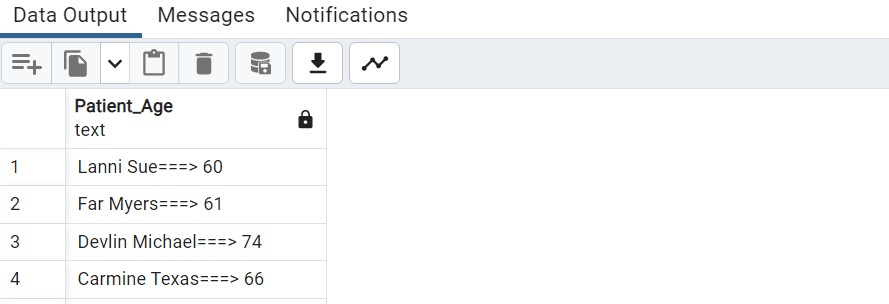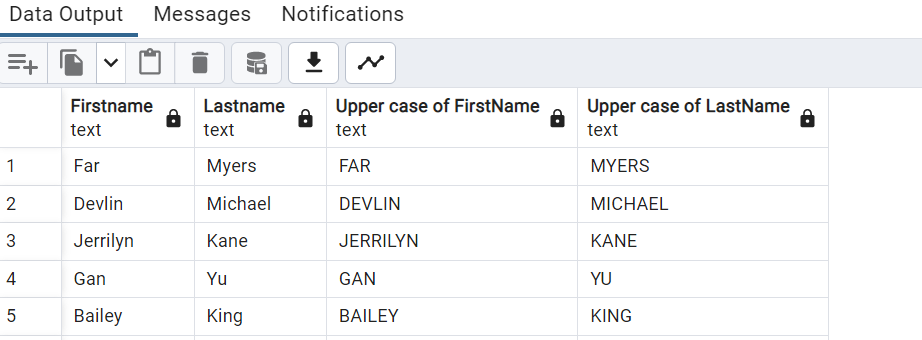top of pageSearch

# Some of the PostgreSQL Functions

1. LENGTH() : The PostgreSQL length() function is used to find the length of a string i.e. number of characters

in the given string.

Example :

SELECT "Patient_ID","Firstname" || ' ' || "Lastname" as "Fullname"

FROM "Patients" WHERE LENGTH( "Firstname" || ' ' || "Lastname") >=15 ;2.RIGHT() : The PostgreSQL right() function is used to extract n number of characters specified in the argument from the right of a given string.

Example:

SELECT Distinct RIGHT("Lab_names",10) AS "Lab_Names"

FROM "Lab_Visit";3. CEILING() / CEIL() : The CEILING() function returns the smallest integer value that is larger

than or equal to a number. CEIL ,It essentially rounds up to the nearest

whole number and is the opposite of the FLOOR function (which

rounds down to the nearest whole number).

The word CEIL is short for CEILING.

Reference Query: SELECT "WBC","RBC" FROM public."Lab_Test" offset 1 limit 5Example:

SELECT CEILING("WBC") AS WBC, CEILING("RBC") AS RBC

FROM PUBLIC."Lab_Test" OFFSET 1 LIMIT 44. FLOOR() : Function returns the largest integer value that is smaller than or equal to a

Number.The purpose of the SQL FLOOR function is to return the highest integer

value that is equal to or less than a number.

Example:

SELECT FLOOR("WBC") AS WBC, FLOOR("RBC") AS RBC

FROM PUBLIC."Lab_Test" OFFSET 1 LIMIT 45. LPAD() : The PostgreSQL lpad() function is used to fill up a string of specific length by

a substring. If the length of the substring is equal to the remaining length of

main string it will fill up properly, if less than the remaining length, the

substring will repeat until it is not filling up, if longer than the remaining length

or specified length it will be truncated on the right.

Example:6. RANK()/ DENSE_RANK(): The DENSE_RANK() is a window function that assigns a rank to

each row within a partition of a result set.However, RANK will then

skip the next available ranking value whereas DENSE_RANK would

still use the next chronological ranking value.

Example:

SELECT "Firstname","Lastname","Age",DENSE_RANK()OVER(

ORDER BY "Age" DESC) AGE_RANK FROM PUBLIC."Patients"7. FETCH() : In PostgreSQL, the FETCH clause is used to fetch/retrieve a specific portion/part of rows returned by any query. An optional clause named OFFSET can be used with the FETCH clause to skip some rows of a table. The FETCH clause performs the same functionality as the LIMIT clause.

Example:

SELECT "Patient_ID","Firstname","Lastname" FROM "Patients"

OFFSET 5 ROWS FETCH NEXT 4 ROWS ONLY;8. LTRIM() : The PostgreSQL ltrim() function is used to remove spaces ( if no

character(s) is provided as trimming_text ) or set of characters which are

matching with the trimming_text, from the start of a string.

Example:

SELECT "Lastname",

LTRIM("Lastname",'Ma') FROM "Patients";9. BTRIM() : The PostgreSQL btrim() function is used to remove the longest string specified

in the argument from the start and end of the given string. If no string for

removing default space will be removed from leading and trailing side from the string.

Example:

SELECT "Firstname", BTRIM("Firstname",'ALan') FROM "Patients"10. REPEAT(): The PostgreSQL repeat() function is used to repeat a specified

string to a specified number of times.

Example:

SELECT CONCAT("Firstname"|| ' ' || "Lastname", REPEAT('=', 3), '> ', "Age") as "Patient_Age"

FROM "Patients";11. CHAR_LENGTH() :The PostgreSQL char_length function or character_length function is used to count the number of characters in a specified string. The CHARACTER_LENGTH() function is similar to CHAR_LENGTH() function.

Example:

SELECT "Lab_names", CHAR_LENGTH("Lab_names") AS "Length of a String"

FROM "Lab_Visit" WHERE "Lab_names" like 'O%' OR "Lab_names" like 'G%' ;12 . LOWER() : The PostgreSQL lower function is used to convert a string from upper case to lower case.

Example:

SELECT DISTINCT "Lab_names", LOWER("Lab_names") FROM "Lab_Visit"

WHERE "Lab_Visit_Date" BETWEEN '2005-09-26' AND '2006-06-12'13 . UPPER() : In PostgreSQL, the UPPER function is used to convert a string into upper case. Like the LOWER function, the UPPER function accepts a string expression or string-convertible expression and converts it to an upper case format.

Example:

SELECT "Firstname","Lastname",

UPPER("Firstname") "Upper case of FirstName",

UPPER("Lastname") "Upper case of LastName"

FROM "Patients" P

JOIN "Gender" G USING("Gender_ID")

WHERE "Gender_ID" = 'G001'14. ARRAY_AGG() : PostgreSQL ARRAY_AGG() function is an aggregate function that accepts a set of values and returns an array where each value in the input set is assigned to an element of the array.

Example:

SELECT P."Patient_ID",

ARRAY_AGG(P."Firstname" || ' ' || P."Lastname" || ' ' || G."Gender" || ' ' || R."Race")

FROM "Patients" P

JOIN PUBLIC."Race" R ON P."Race_ID" = R."Race_ID"

JOIN PUBLIC."Gender" G ON P."Gender_ID" = G."Gender_ID"

GROUP BY P."Patient_ID"15 . LEFT() : The PostgreSQL left() function is used to extract n number of characters specified in the argument from the left of a given string. When the value of n is negative, the extraction will happen from left except last n characters.

Example:

SELECT Distinct LEFT("Lab_names",10) AS "Lab_Names"

FROM "Lab_Visit";16. EXPLAIN() : The EXPLAIN shows how tables involved in a statement will be scanned by index scan or sequential scan

Example :

EXPLAIN SELECT Distinct LEFT("Lab_names",10) AS "Lab_Names"

FROM "Lab_Visit";17 . ORDER BY RANDOM() : PostgreSQL order by the random function is used to return the random number from the table by using the order by clause. Random function with an order by clause will not work the same as the order by clause in PostgreSQL because the random function will pick the random values from the table in PostgreSQL.

Example:

SELECT SUBSTRING("Patient_ID",3,5) AS "pat_id",

SUBSTRING("Patient_ID",3,5) ::integer % 2 = 0 AS EVEN,

SUBSTRING("Patient_ID",3,5) ::integer % 2 <> 0 AS ODD

FROM "Patients"

ORDER BY RANDOM();18 . POSITION() : The PostgreSQL position function is used to find the location of a substring within a specified string.

Example:

SELECT "Patient_ID", "Lastname",

POSITION('an' in "Lastname") "Position of 'an' in Lastname"

FROM "Patients"

WHERE SUBSTRING("Lastname",POSITION('an' in "Lastname"),2) = 'an' ;19 . SUM() : The SUM function in PostgreSQL returns the sum of values for the list of selected columns.

Example:

SELECT SUM("Diabetes_Duration") FROM "Patients" A

WHERE A."Group_ID" = 'GRP_02';20 . ROUND() : The PostgreSQL round() function is used to return the value after rounded a number upto a specific decimal places, provided in the argument.

Example:

SELECT "Lab_names",

ROUND(COUNT(*) * 100.0 / SUM(COUNT(*))OVER()) PERCENTAGE

FROM "Lab_Visit"

GROUP BY "Lab_names";